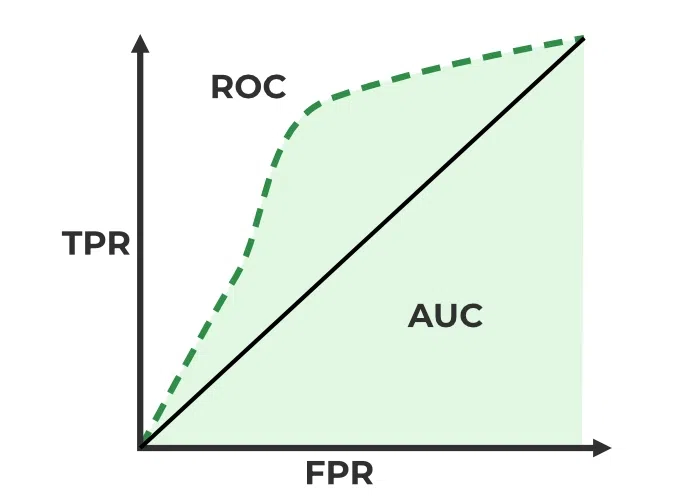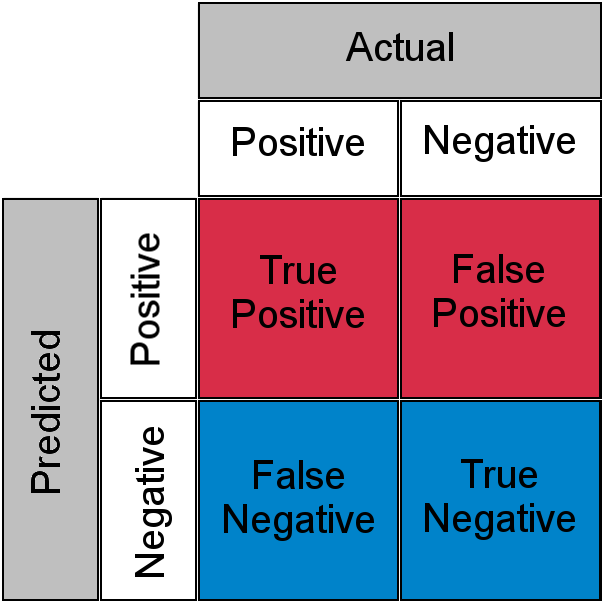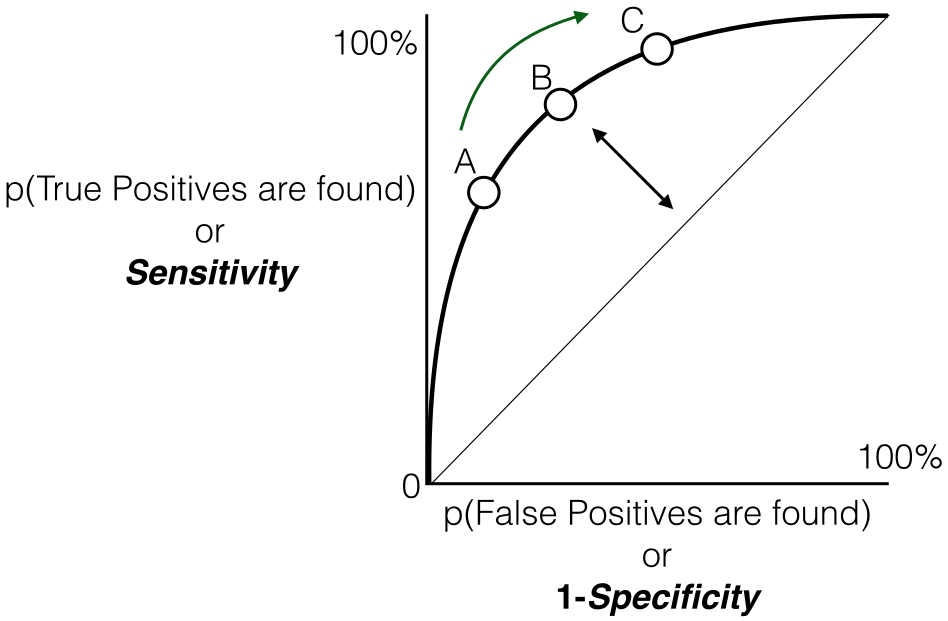# Guide to AUC ROC Curve in Machine Learning

One important aspect of Machine Learning is model evaluation. You need to have some mechanism to evaluate your model. This is where these performance metrics come into the picture they give us a sense of how good a model is. If you are familiar with some basics of Machine Learning then you must have across some of these metrics like accuracy, precision, recall, auc-roc, etc which are generally used for classification tasks. In this article, we will explore in depth the one such metrics which is the AUC-ROC curve.

## AUC-ROC curve

Let’s first understand the meaning of the two terms ROC and AUC

• AUC: Area Under Curve

### ROC Curve

ROC stands for Receiver Operating Characteristics, and the ROC curve is the graphical representation of the effectiveness of the binary classification model. It plots the true positive rate (TPR) vs the false positive rate (FPR) at different classification thresholds.

### AUC Curve:

AUC stands for Area Under the Curve, and the AUC curve represents the area under the ROC curve. It measures the overall performance of the binary classification model. As both TPR and FPR range between 0 to 1, So, the area will always lie between 0 and 1, and A greater value of AUC denotes better model performance. Our main goal is to maximize this area in order to have the highest TPR and lowest FPR at the given threshold. The AUC measures the probability that the model will assign a randomly chosen positive instance a higher predicted probability compared to a randomly chosen negative instance.

It represents the probability with with our model is able to distinguish between the two classes which are present in our target.ROC-AUC Classification Evaluation Metric

## TPR and FPR

This is the most common definition that you would have encountered when you would Google AUC-ROC. Basically, the ROC curve is a graph that shows the performance of a classification model at all possible thresholds( threshold is a particular value beyond which you say a point belongs to a particular class). The curve is plotted between two parameters

• TPR – True Positive Rate
• FPR – False Positive Rate

Before understanding, TPR and FPR let us quickly look at the confusion matrix.Confusion Matrix for a Classification Task

• True Positive: Actual Positive and Predicted as Positive
• True Negative: Actual Negative and Predicted as Negative
• False Positive(Type I Error): Actual Negative but predicted as Positive
• False Negative(Type II Error): Actual Positive but predicted as Negative

In simple terms, you can call False Positive a false alarm and False Negative a miss. Now let us look at what TPR and FPR are.

### Sensitivity / True Positive Rate / Recall

Basically, TPR/Recall/Sensitivity is the ratio of positive examples that are correctly identified.  It represents the ability of the model to correctly identify positive instances and is calculated as follows:Sensitivity/Recall/TPR measures the proportion of actual positive instances that are correctly identified by the model as positive.

### False Positive Rate

FPR is the ratio of negative examples that are incorrectly classified.Specificity measures the proportion of actual negative instances that are correctly identified by the model as negative. It represents the ability of the model to correctly identify negative instances

And as said earlier ROC is nothing but the plot between TPR and FPR across all possible thresholds and AUC is the entire area beneath this ROC curve.Sensitivity versus False Positive Rate plot

## How does AUC-ROC work?

We looked at the geometric interpretation, but I guess it is still not enough in developing the intuition behind what 0.75 AUC actually means, now let us look at AUC-ROC from a probabilistic point of view. Let us first talk about what AUC does and later we will build our understanding on top of this

AUC measures how well a model is able to distinguish between classes.

An AUC of 0.75 would actually mean that let’s say we take two data points belonging to separate classes then there is a 75% chance the model would be able to segregate them or rank order them correctly i.e positive point has a higher prediction probability than the negative class. (assuming a higher prediction probability means the point would ideally belong to the positive class). Here is a small example to make things more clear.

Here we have 6 points where P1, P2, and P5 belong to class 1 and P3, P4, and P6 belong to class 0 and we’re corresponding predicted probabilities in the Probability column, as we said if we take two points belonging to separate classes then what is the probability that model rank orders them correctly.

We will take all possible pairs such that one point belongs to class 1 and the other belongs to class 0, we will have a total of 9 such pairs below are all of these 9 possible pairs.

Here column isCorrect tells if the mentioned pair is correctly rank-ordered based on the predicted probability i.e class 1 point has a higher probability than class 0 point, in 7 out of these 9 possible pairs class 1 is ranked higher than class 0, or we can say that there is a 77% chance that if you pick a pair of points belonging to separate classes the model would be able to distinguish them correctly. Now, I think you might have a bit of intuition behind this AUC number, just to clear up any further doubts let’s validate it using Scikit learns AUC-ROC implementation.

## Python3

 import numpy as np from sklearn .metrics import roc_auc_score   y_true = [1, 1, 0, 0, 1, 0] y_pred = [0.95, 0.90, 0.85, 0.81, 0.78, 0.70] auc = np.round(roc_auc_score(y_true, y_pred), 3) print("Auc for our sample data is {}".format(auc))

Output:

Auc for our sample data is 0.778

## When should we use the AUC-ROC evaluation metric?

Having said that there certain places where ROC-AUC might not be ideal. ROC-AUC does not work well under severe imbalance in the dataset, to give some intuition for this let us look back at the geometric interpretation here. Basically, ROC is the plot between TPR and FPR( assuming the minority class is a positive class), now let us have a close look at the FPR formula againThe denominator of FPR has True Negatives as one factor since the Negative Class is in the majority the denominator of FPR is dominated by True Negatives which makes FPR less sensitive to any changes in minority class predictions. To overcome this, Precision-Recall Curves are used instead of ROC and then the AUC is calculated, try to answer this yourself how does the Precision-Recall curve handle this problem?

Hint: Recall and TPR are the same technically only FPR is replaced with Precision, just compare the denominators for both and try to assess how the imbalance problem is solved here.

ROC-AUC tries to measure if the rank ordering of classifications is correct it does not take into account actually predicted probabilities, let me try to make this point clear with a small code snippet.

## Python3

 import pandas as pd   y_pred_1 = [0.99, 0.98, 0.97, 0.96,             0.91, 0.90, 0.89, 0.88] y_pred_2 = [0.99, 0.95, 0.90, 0.85,             0.20, 0.15, 0.10, 0.05] y_act = [1, 1, 1, 1, 0, 0, 0, 0] test_df = pd.DataFrame(zip(y_act, y_pred_1,                            y_pred_2),                        columns=['Class',                                 'Model_1', 'Model_2']) test_df

Output:

   Class  Model_1  Model_2
0      1     0.99     0.99
1      1     0.98     0.95
2      1     0.97     0.90
3      1     0.96     0.85
4      0     0.91     0.20
5      0     0.90     0.15
6      0     0.89     0.10
7      0     0.88     0.05

So ideally one should use AUC when their dataset does not have a severe imbalance and when your use case does not require you to use actually predicted probabilities.

## How to use ROC-AUC for a multi-class model?

For a multi-class setting, we can simply use one vs all methodology and you will have one ROC curve for each class. Let’s say you have four classes A, B, C, and D then there would be ROC curves and corresponding AUC values for all the four classes, i.e. once A would be one class and B, C, and D combined would be the others class, similarly, B is one class and A, C, and D combined as others class, etc.

Whether you're preparing for your first job interview or aiming to upskill in this ever-evolving tech landscape, GeeksforGeeks Courses are your key to success. We provide top-quality content at affordable prices, all geared towards accelerating your growth in a time-bound manner. Join the millions we've already empowered, and we're here to do the same for you. Don't miss out - check it out now!

Previous
Next